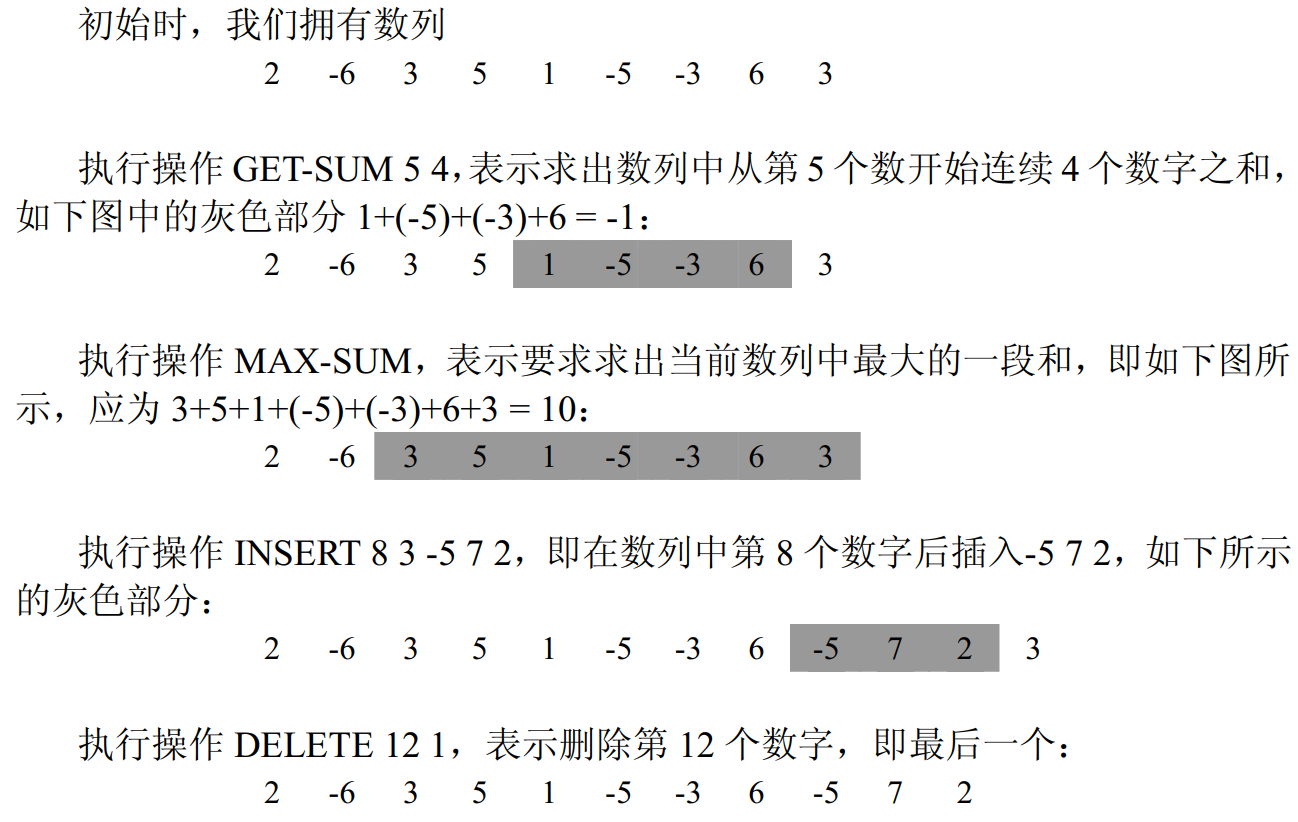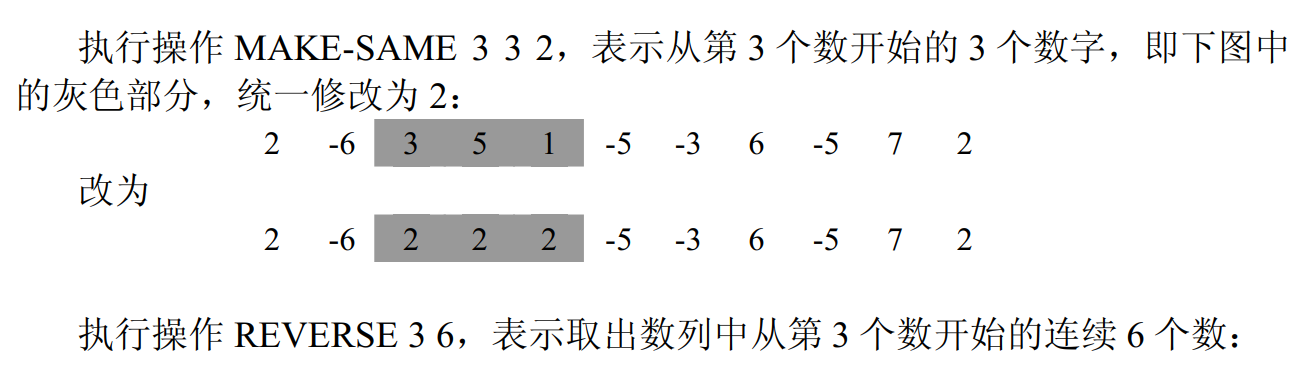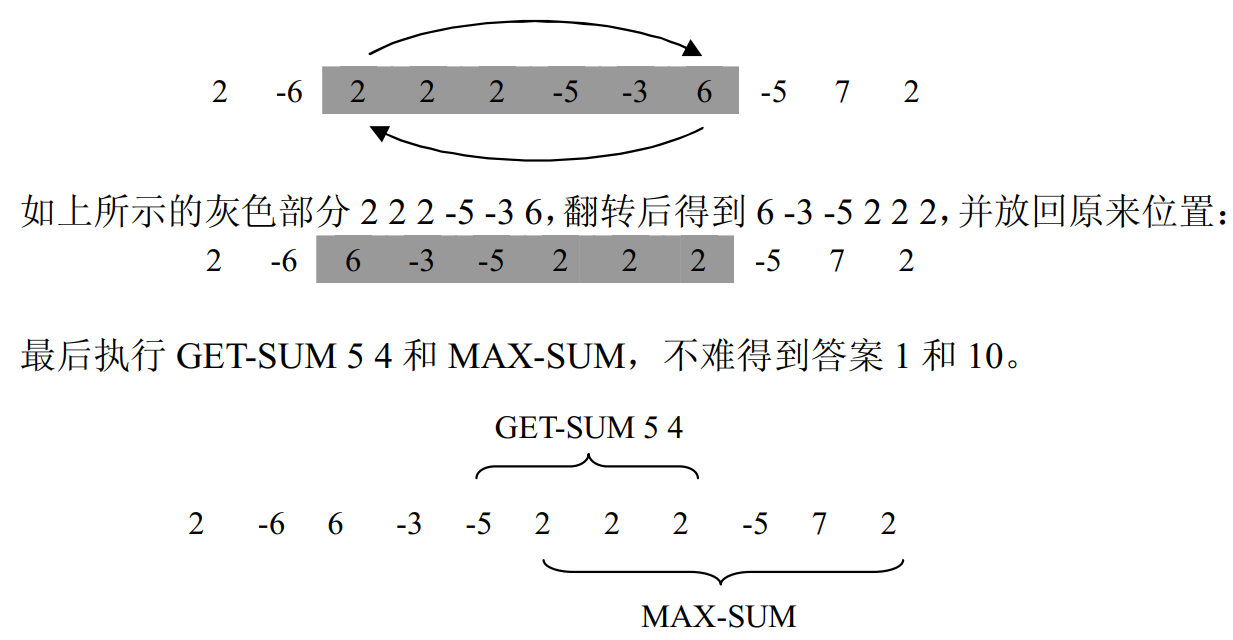# 洛谷 P2042 [NOI2005]维护数列 题解【平衡树】

一道令人闻风丧胆的平衡树题终于过了……

## 题目描述

 操作编号 输入文件中的格式 说明 1.插入 $INSERT\_pos_i\_tot\_c_1\_c_2\_\dots \_c_{tot}$ 在当前数列的第$pos_i$个数字后插入$tot$个数字：$c_1,c_2,\dots,c_{tot}$；若在数列首插入，则$pos_i$为$0$ 2.删除 $DELETE\_pos_i\_tot$ 从当前数列的第$pos_i$个数字开始连续删除$tot$个数字 3.修改 $MAKE-SAME\_pos_i\_tot\_c$ 将当前数列的第$pos_i$个数字开始的连续$tot$个数字统一修改为$c$ 4.翻转 $REVERSE\_pos_i\_tot$ 取出从当前数列的第$pos_i$个数字开始的$tot$个数字，翻转后放入原来的位置 5.求和 $GET-SUM\_pos_i\_tot$ 计算从当前数列开始的第$posi$个数字开始的$tot$个数字的和并输出 6.求和最大的子列 $MAX-SUM$ 求出当前数列中和最大的一段子列，并输出最大和

## 输入输出样例

9 8
2 -6 3 5 1 -5 -3 6 3
GET-SUM 5 4
MAX-SUM
INSERT 8 3 -5 7 2
DELETE 12 1
MAKE-SAME 3 3 2
REVERSE 3 6
GET-SUM 5 4
MAX-SUM

-1
10
1
10

## 说明• 如果你的程序能在输出文件正确的位置上打印 GET-SUM 操作的答案，你可以得到该测试点$60\%$的分数；
• 如果你的程序能在输出文件正确的位置上打印 MAX-SUM 操作的答案，你可以得到该测试点$40\%$的分数；
• 以上两条的分数可以叠加，即如果你的程序正确输出所有 GET-SUM MAX-SUM 操作的答案，你可以得到该测试点$100\%$的分数。

$50\%$的数据中，任何时刻数列中最多含有 30 000 个数；
$100\%$的数据中，任何时刻数列中最多含有 500 000 个数。
$100\%$的数据中，任何时刻数列中任何一个数字均在[-1 000, 1 000]内。
$100\%$的数据中，$M≤$20 000，插入的数字总数不超过 4 000 000 个，输入文件大小不超过 20MBytes

## 题解：

这个题几乎包含了平衡树的所有操作（不过没有维护父节点？），同时还要求空间回收。

我是用fhq_treap实现的，常数可能稍微大了点，不过NOIp应该够用了。这时平衡树维护的关键字是顺序而不是权值，和文艺平衡树一题有些类似，但是这个题维护的东西要多一些。

插入就直接暴力插入吧，笛卡尔树也许能起到一点优化效果，但是还是要进行Merge操作的。删除和一般的平衡树删除操作类似，直接把要删除的部分抽出来，然后合并两边即可。修改时就需要lazytag了，注意每次树的形态变化时都要pushdown和maintain一次，这样是最不容易出错的。

翻转和文艺平衡树就一模一样了，需要一个额外的lazytag，每次翻转给这个lazytag xor 1就可以了，pushdown的时候如果是1就旋转左右儿子。求和也比较好做，在维护size的同时加一下就好了，重点是维护最大子段和。

最大子段和在线段树中的维护已经写过几遍了，一开始感觉平衡树也差不多，但是平衡树除了左右儿子还多了一个根节点，这样就比较麻烦。不过在线段树中要维护的，在这里还是要维护。比如说最大前缀、最大后缀，除了要多考虑自己以外都和线段树一模一样。实际上，我们可以把自己归为左儿子，不过我没有这样实现，因为本身多考虑一层层次也比较清晰，感觉自己看得清楚就可以。

不过还有几个问题，比如旋转后前后缀交换的问题。这里更新只关系到了左右儿子，那么把左右儿子翻转后，对它们分别做pushdown，这样从下面更新就不会影响了。同时要注意maintain和pushdown要分开，不然pushdown就是$O(n)$的了。对于无论有没有把自己归为左儿子或，都会有左儿子或右儿子为空的情况，这时把它看作0即可，因为此时的左端点或右端点就是自己，防止了统计答案出错。

同时，在上面的操作基础上，会出现整段为负而保存的值为0的情况。那么此时的最大子段和就是整个数列中的最大值，那么我们额外维护一下最大值就可以了。当查询答案发现为0时，就可以直接输出最大值。因为最大值一定小于等于0。

感觉这个题的层次梳理出来了，但是常数比较大，这个其实没关系，fhqtreap的特性。不过要注意这个题卡空间，全程可能影响的点有$4\times 10^6$个，那么对于指针来说我们可以用delete删点，不过这个操作是比较暴力的，需要遍历子树来删，相当于用时间换空间。

## Code：

#include<cstdio>
#include<cstring>
#include<cstdlib>
#include<algorithm>
using std::max;
using std::min;
int L,R,ll,rr,lm,rm,lS,rS;
struct node
{
int sum,sz;
short rdm,rev;
int lazy;
int mx,mxx,l,r,key;
//最大子段，最大权值
node *ls,*rs;
node(int key)
{
this->key=key;
rdm=rand();
sz=1,sum=key;
mx=key>0?key:0;
l=mx,r=mx;
mxx=key;
rev=0,lazy=6666;
ls=NULL;
rs=NULL;
}
node()
{
ls=NULL;
rs=NULL;
}
void pushdown()
{
if(rev)
{
rev=0;
if(ls)
ls->rev^=1;
if(rs)
rs->rev^=1;
node *tmp=ls;
ls=rs;
rs=tmp;
int t=l;
l=r;
r=t;
}
if(lazy!=6666)
{
int x=lazy;
lazy=6666;
if(ls)
ls->lazy=x;
if(rs)
rs->lazy=x;
key=x;
sum=key*sz;
mxx=key;
if(x>0)
{
mx=sum;
l=sum;
r=sum;
}
else
{
l=0,r=0;
mx=0;
}
}
}
void maintain()//共需要维护6个值
{
if(ls)
ls->pushdown();
if(rs)
rs->pushdown();
L=(ls?ls->r:0);
R=(rs?rs->l:0);
ll=(ls?ls->l:0);
rr=(rs?rs->r:0);
lm=(ls?ls->mx:0);
rm=(rs?rs->mx:0);
lS=(ls?ls->sum:0);
rS=(rs?rs->sum:0);
sz=(ls?ls->sz:0)+1+(rs?rs->sz:0);
sum=lS+key+rS;
mxx=max(key,max(ls?ls->mxx:-100000,rs?rs->mxx:-100000));
mx=max(max(lm,rm),key);
mx=max(L+key+R,mx);
l=max(ll,lS+key+R);
r=max(rr,rS+key+L);
}

}*root=NULL;
node *Merge(node *a,node *b)
{
if(!a||!b)
return a?a:b;
a->pushdown();
b->pushdown();
if(a->rdm<b->rdm)
{
a->rs=Merge(a->rs,b);
a->maintain();
return a;
}
b->ls=Merge(a,b->ls);
b->maintain();
return b;
}
void split(node *rt,int x,node *&a,node *&b)
{
if(!rt)
{
a=NULL;
b=NULL;
return;
}
rt->pushdown();
int l=rt->ls?rt->ls->sz:0;
if(l+1<=x)
{
a=rt;
split(a->rs,x-l-1,a->rs,b);
}
else
{
b=rt;
split(b->ls,x,a,b->ls);
}
rt->maintain();
}
void Delete(node *&rt)
{
if(rt->ls)
Delete(rt->ls);
if(rt->rs)
Delete(rt->rs);
delete(rt);
}
char op;
int main()
{
srand(123);
int n,m,u,v,w;
scanf("%d%d",&n,&m);
for(int i=1;i<=n;++i)
{
scanf("%d",&u);
root=Merge(root,new node(u));
}
for(int i=1;i<=m;++i)
{
scanf("%s",op);
if(op=='I')
{
node *New=NULL;
scanf("%d%d",&u,&w);
for(int j=1;j<=w;++j)
{
scanf("%d",&v);
New=Merge(New,new node(v));
}
node *a,*b;
split(root,u,a,b);
root=Merge(Merge(a,New),b);
}
else if(op=='D')
{
node *a,*b,*c;
scanf("%d%d",&u,&v);
split(root,u-1,a,b);
split(b,v,b,c);
root=Merge(a,c);
Delete(b);
}
else if(op=='R')
{
node *a,*b,*c;
scanf("%d%d",&u,&v);
split(root,u-1,a,b);
split(b,v,b,c);
b->rev^=1;
root=Merge(Merge(a,b),c);
}
else if(op=='G')
{
node *a,*b,*c;
scanf("%d%d",&u,&v);
if(!v)
{
printf("%d\n",0);
continue;
}
split(root,u-1,a,b);
split(b,v,b,c);
b->pushdown();
printf("%d\n",b->sum);
root=Merge(Merge(a,b),c);
}
else if(op=='K')
{
node *a,*b,*c;
scanf("%d%d%d",&u,&v,&w);
split(root,u-1,a,b);
split(b,v,b,c);
b->lazy=w;
root=Merge(Merge(a,b),c);
}
else
{
root->pushdown();
printf("%d\n",root->mx?root->mx:root->mxx);
}
}
return 0;
}

### 说点什么Subscribe

/* */